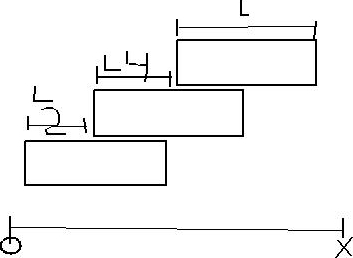# Problem: find the x coordinate of the center of mass of the bricks shown inthe figure.

###### FREE Expert Solution

In this problem we are working out the x-coordinate of the center of mass.

X-coordinate of the center of mass:

$\overline{){{\mathbf{x}}}_{\mathbf{c}\mathbf{m}}{\mathbf{=}}\frac{{\mathbf{m}}_{\mathbf{1}}{\mathbf{x}}_{\mathbf{1}}\mathbf{+}{\mathbf{m}}_{\mathbf{2}}{\mathbf{x}}_{\mathbf{2}}\mathbf{+}\mathbf{.}\mathbf{.}\mathbf{.}\mathbf{+}{\mathbf{m}}_{\mathbf{n}}{\mathbf{x}}_{\mathbf{n}}}{{\mathbf{m}}_{\mathbf{1}}\mathbf{+}{\mathbf{m}}_{\mathbf{2}}\mathbf{+}\mathbf{.}\mathbf{.}\mathbf{.}\mathbf{+}{\mathbf{m}}_{\mathbf{n}}}}$

89% (284 ratings)###### Problem Details

find the x coordinate of the center of mass of the bricks shown inthe figure.Frequently Asked Questions

What scientific concept do you need to know in order to solve this problem?

Our tutors have indicated that to solve this problem you will need to apply the Center of Mass & Simple Balance concept. You can view video lessons to learn Center of Mass & Simple Balance. Or if you need more Center of Mass & Simple Balance practice, you can also practice Center of Mass & Simple Balance practice problems.

What professor is this problem relevant for?

Based on our data, we think this problem is relevant for Professor Hendrickson's class at University of Wisconsin - Eau Claire.